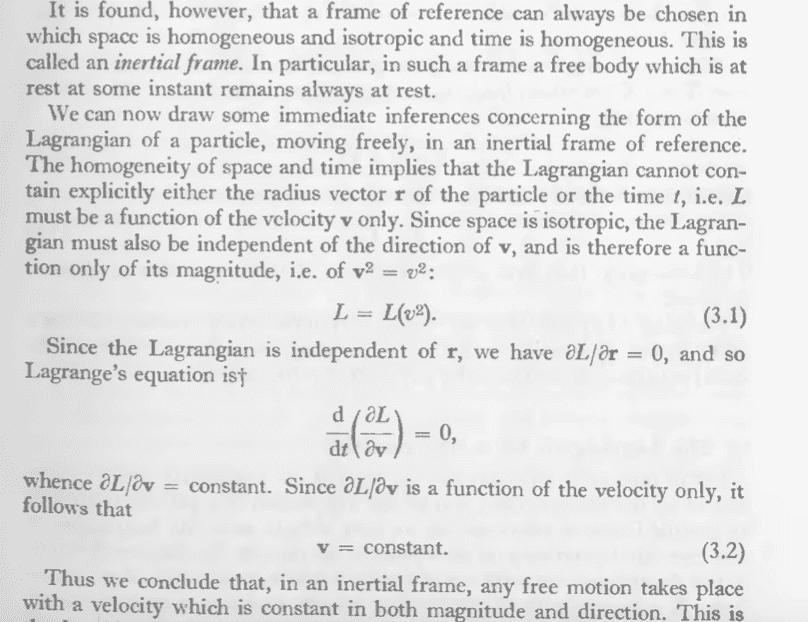# On Landau vol.1 Pg.5 (Question about conclusion drawn by Landau)

zhuang382
Homework Statement:
How do we deduce the conclusion of (3,2)? This is a topic inertial frame.
Relevant Equations:
Law of inertia
I understand that d/dv(L) = constant, and L is only dependent on v, but how do we get to the fact that v = constant?Gold Member
Say
$$\frac{\partial L}{\partial v}=f(v)$$,

$$\frac{d}{dt}f(v)= \frac{df(v)}{dv}\frac{dv}{dt}=0$$

Usually ##\frac{df(v)}{dv} \neq 0## so

$$\frac{dv}{dt}=0$$

•zhuang382
zhuang382
Thanks!

•anuttarasammyak